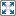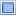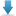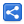# Java Regex - Capturing Groups

[Last Updated: Apr 28, 2017]

We can combine individual or multiple regular expressions as a single group by using parentheses `()`. These groups can serve multiple purposes. In our basic tutorial, we saw one purpose already, i.e. alteration using logical OR (the pipe '|'). Other than that groups can also be used for capturing matches from input string for expression.

The groups are assigned a number by the regex engine automatically. It happens at the time when a match is found. Consider the regex pattern "([A-Z])([0-9])". It has two groups. ([A-Z]) will be assigned the number 1 and ([0-9]) will be assigned the number 2. There's always a special group number zero which represents the entire match. Followings are useful methods of `Matcher` class to get the information related to capturing groups.

1. `String Matcher#group()`: returns the recent match. The match is grouped as number 0. The returned string is equivalent to `s.substring(matcher.start(), matcher.end())`, where "s" is the entire input string.
2. `int Matcher#start()`: The start index of the recent match. This match is captured as group number 0.
3. `int Matcher#end()`: The end index of recent match. This match is captured as group number 0.
4. `String group(int n)`: returns the substring captured by group number 'n'.
Calling `matcherInstnce.group(0)` is equivalent to calling `matcherInstnce.group()`
5. `int Matcher#start(int n)`: returns the start index of captured group number 'n'.
6. `int Matcher#end(int n)`: returns the end index of captured group number n.
7. `int Matcher#groupCount()`: returns the number of groups captured in the recent match. Group zero denotes the entire recent match. Also it's important to know that, the captured group numbering starts from 1. Don't confuse this number with zero based index like in arrays, it is just a group number or more accurately, it is just a group identity. In case if we want to run a for-loop through all captured groups, our loop should start from 1 and should end at `Matcher#groupCount()` inclusively.

#### Example

Assume we have some text data which has all cities phone area codes, given by a consistent format:

`Birmingham AL: 205, Baytown TX: 281, Chapel Hill NC: 284, .......`

Let's say we want to extract individual city names along with state codes and area codes. Let's construct our regex:

```   String regex = "\\b[A-Za-z\\s]+,\\s[A-Z]{2,2}:\\s[0-9]{3,3}\\b";
String input = "This is the list: Baytown, TX: 281, Chapel Hill, NC: 284, " +
"Fort Myers, FL: 239";

Pattern pattern = Pattern.compile(regex);
Matcher matcher = pattern.matcher(input);

int matchCount = 0;
while (matcher.find()) {
matchCount++;
System.out.printf("Match count: %s, Group Zero Text: '%s'%n", matchCount,
matcher.group());
for (int i = 1; i < matcher.groupCount(); i++) {
System.out.printf("Capture Group Number: %s, Captured Text: '%s'%n", i,
matcher.group(i));
}
}```

Here's the explanation of our regex pattern:

• `[A-Za-z\\s]+`: specifies city name. It's not expecting any character other than alphabets or white-spaces between the words. The '+' at the end specifies that city name length should be of one or more characters long.
• `[A-Z]{2,2}`: specifies exactly two capital letters for state code.
• `[0-9]{3,3}`: specifies exactly three digits for phone area code.
• Notice `\\b` at the beginning and at the end for the word boundary to avoid white-spaces spaces around each match. There are also two literals `,` and `:`
`\\s` for a fixed white-space.

Output;

```Match count: 1, Group Zero Text: 'Baytown, TX: 281'
Match count: 2, Group Zero Text: 'Chapel Hill, NC: 284'
Match count: 3, Group Zero Text: 'Fort Myers, FL: 239'```

We don't see any output from inside the for loop yet. That's because we haven't defined any capturing groups `()`. Let's add capturing groups `()` around city name, state code and phone area code.

```   String regex = "\\b([A-Za-z\\s]+),\\s([A-Z]{2,2}):\\s([0-9]{3,3})\\b";
String input = "This is the list: Baytown, TX: 281, Chapel Hill, NC: 284, "+
"Fort Myers, FL: 239";

Pattern pattern = Pattern.compile(regex);
Matcher matcher = pattern.matcher(input);

int matchCount = 0;
while (matcher.find()) {
matchCount++;
System.out.printf("Match count: %s, Group Zero Text: '%s'%n", matchCount,
matcher.group());
for (int i = 1; i <= matcher.groupCount(); i++) {
System.out.printf("Capture Group Number: %s, Captured Text: '%s'%n", i,
matcher.group(i));
}
}```

Output:

```Match count: 1, Group Zero Text: 'Baytown, TX: 281'
Capture Group Number: 1, Captured Text: 'Baytown'
Capture Group Number: 2, Captured Text: 'TX'
Match count: 2, Group Zero Text: 'Chapel Hill, NC: 284'
Capture Group Number: 1, Captured Text: 'Chapel Hill'
Capture Group Number: 2, Captured Text: 'NC'
Match count: 3, Group Zero Text: 'Fort Myers, FL: 239'
Capture Group Number: 1, Captured Text: 'Fort Myers'
Capture Group Number: 2, Captured Text: 'FL'
```

We successfully extracted the desired values. There's one problem though. Iterating though the captured groups via 'for loop', cannot help us to distinguish between the three captured values. Instead, we have to explicitly call `matcher.group(desiredGroupNumber)`. That's a safe way to do that, because the time we construct regex pattern along with capturing groups, we already know the group number. Let's modify our example one more time. In practice we actually be populating some object with extracted information. That's the way we can freely use that information throughout our application.

```    private final static String regex = "\\b([A-Za-z\\s]+),\\s([A-Z]{2,2}):\\s([0-9]{3,3})\\b";
private final static Pattern pattern = Pattern.compile(regex);

public void showAreaCodes(String textData) {
List <PhoneAreaCode> areaCodeList = getAreaCodeList(textData);
//do whatever we want to do with area code list
}

public <PhoneAreaCode> getAreaCodeList(String textData) {
<PhoneAreaCode> areaCodeList = new ArrayList<PhoneAreaCode>();
Matcher matcher = pattern.matcher(textData);

while (matcher.find()) {
if (matcher.groupCount() == 3) {
new PhoneAreaCode(matcher.group(1), matcher.group(2),
matcher.group(3)));
}
}
return areaCodeList;
}```

#### Example Project

Dependencies and Technologies Used:

• JDK 1.8
• Maven 3.0.4

 Regex Capturing GroupsSelect AllDownload• regex-capturing-groups
• src
• main
• java
• com
• logicbig
• example
• WithGroups.java

 Share